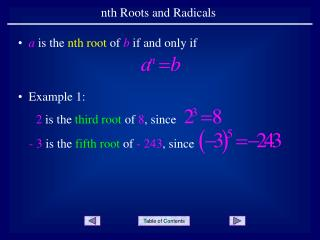DownloadDownload PresentationDownload Presentation- - - - - - - - - - - - - - - - - - - - - - - - - - - E N D - - - - - - - - - - - - - - - - - - - - - - - - - - -
##### Presentation Transcript

1. 2 is the third root of 8, since - 3 is the fifth root of -243, since nth Roots and Radicals • a is the nth root of b if and only if • Example 1:

2. 3 is the fourth root of 81, since - 3 is also the fourth root of 81, since • As we saw with square roots, when n is even, there can be two possible nth roots. • Example 2:

3. Sometimes there are nonthroots of a number. • Example 3: There are no fourth roots of – 16 since there are no numbers such that Note that neither2 nor – 2 will work.

4. is called a radical symbol is called a radical • A common way to express nth roots is using radical notation. b is called the radicand n is called the index

5. Example 4 32 is the radicand 5 is the index

6. Example 5 36 is the radicand Since there is no number written in the index position, it is assumed to be a2, or square root.

7. and • Since both 2 and -2 are fourth roots of 16. • Expressed in radical notation … Read this as the fourth root of 16 is 2. Note that we use only the positive 2 and not the negative 2.

8. Consider the radical: • As with square roots, when n is even and radical notation is used, the result is the principalnth root of b, which is the non-negative root. • Example 6 There are two fourth roots of 81, - 3 and 3. The principal fourth root is the non-negative root, or 3. Therefore,

9. Consider the radical: • Example 7 With an odd index, there is only one third root of – 8. Therefore,

10. Evaluation Reasoning • Example 8

11. Evaluation Reasoning • Example 9

12. As shown in previous slide shows, there are special ways to describe radicals when the index is either a 2 or a 3. Index = 2: Square Root Index = 3: Cube Root • Example 10 Square root of 9 Cube root of 27

13. END OF PRESENTATION Click to rerun the slideshow.